I thought I would share one of my favorite constructions in Haskell, namely that adjoint functors give rise to monads. Although it’s a trivial result in category theory how it manifests in Haskell is quite lovely.

A Functor in Haskell maps objects and morphism (i.e. functions) in a subcategory of Hask to objects and morphisms of another category.

class Functor f where
fmap :: (a -> b) -> f a -> f b

And satisfies the functor laws:

fmap id = id
fmap (a . b) = (fmap a) . (fmap b)

In commutative diagrams we draw objects as points and morphisms as arrows. In a string diagrams we invert this and draw morphisms as points and objects as lines.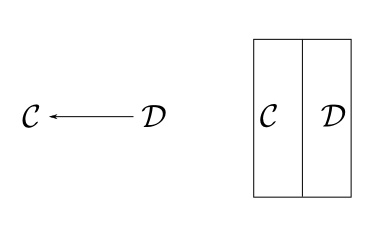Functor composition is defined for $$F : \mathcal{A} \rightarrow \mathcal{B}$$, $$G : \mathcal{B} \rightarrow \mathcal{C}$$ as $$G \circ F : \mathcal{A} \rightarrow \mathcal{C}$$, and is drawn with parallel lines.

newtype FComp f g a = C { unC :: f (g a) }

instance (Functor f, Functor g) => Functor (FComp f g) where
fmap f (C x) = C (fmap (fmap f) x)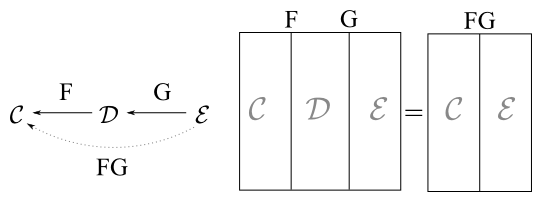Generally the composition of functors $$F \circ G$$ is written simply as $$FG$$. Composition diagrammatically allows us to collapse adjacent segments in our string diagram.

The identity functor ( $$\text{Id}$$ ) is the functor that maps each morphism and object to itself.

newtype Id a = Identity { unId :: a }

instance Functor Id where
fmap f x = Identity (f (unId x))

Composition with the identity functor forms an identity relation:

$F \circ \text{Id}_B = F \\ \text{Id}_A \circ F = F$

As witnessed by the expressions:

left :: Functor f => FComp f Id a -> f a
left (C a) = fmap unId a

right :: Functor f => f a -> FComp f Id a
right a = C \$ fmap Identity a

We’ll follow the convention to omit the identity functor, and it is shown as a dotted line in subsequent string diagrams.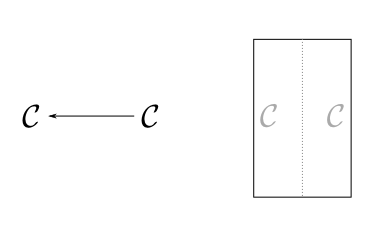A natural transformation in our context will be a polymorphic function associated with two Haskell functor instances f and g with type signature (Functor f, Functor g) => forall a. f a -> g a. Which could be written with the following type synonym.

type Nat f g = forall a. f a -> g a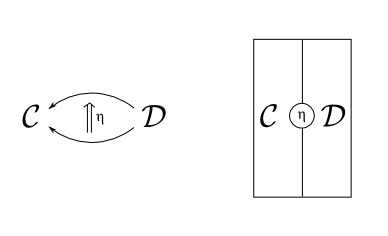The identity natural transform mapping a functor $$F$$ to itself is written $$1_F$$ and in Haskell is just (id). The composition of natural transformations follows the associativity laws, shown below: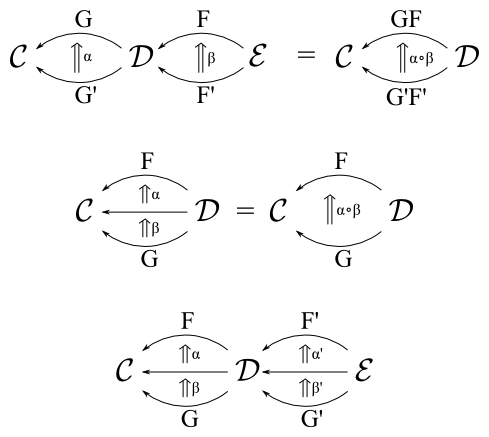The final interchange law states that we can chase the natural transformations through the functors horizontally or compose natural transformation between functors vertically and still arrive at the same result.

$(\alpha \beta) \circ (\alpha' \beta') = (\alpha \alpha') \circ (\beta \beta')$

type NatComp f f' g g' = forall a. f' (f a) -> g' (g a)

vert :: (Functor f, Functor f', Functor g, Functor g') =>
Nat f' g' -> Nat f g -> NatComp f f' g g'
vert a b x = a (fmap b x)

horiz :: (Functor f, Functor f', Functor g, Functor g') =>
Nat f' g' -> Nat f g -> NatComp f f' g g'
horiz a b x = fmap b (a x)

By the interchange law horiz and vert must be interchangable under composition. For natural transformations a, b, a', b' in Haskell we have the equation:

(a . b) vert (a' . b') == (a horiz a') . (b horiz b')

A diagram example for a natural transformation $$\eta : 1_\mathcal{C} \rightarrow {FG}$$ between the identity functor and the composition functor of $$FG$$ would be drawn as: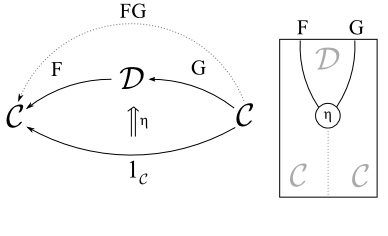An isomorphism $$F \cong G$$ implies that composition of functors is invertible in that $$F G = \text{Id}_C$$ and $$G F = \text{Id}_D$$. An adjoint $$F ⊣ G$$ between a pair of functors $$F : D \rightarrow C$$ and $$G : C \rightarrow D$$ is a weaker statement that there exists a pair of associated natural transformations $$(F, G, \epsilon, \eta)$$ with:

$\epsilon : FG \rightarrow 1_\mathcal{C} \\ \eta : 1_\mathcal{D} \rightarrow FG$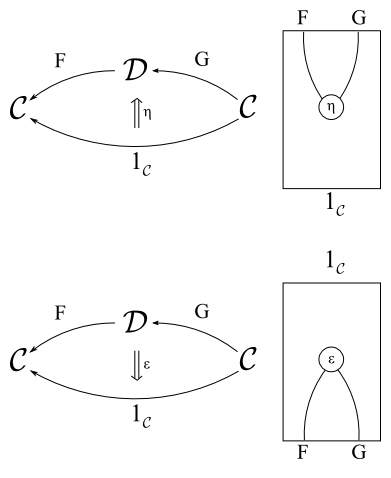Such that the following triangle identities hold:

$(\epsilon F) \circ (F \eta) = 1_F \\ (G \epsilon) \circ (\eta G) = 1_G$

These are drawn below: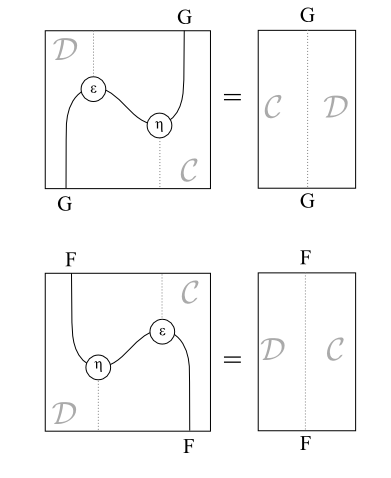In terms of the categories $$C,D$$ an adjoint is in some sense a “half-isomorphism” or “almost inverse” but some structure is lost in one direction.

$$\eta$$ and $$\epsilon$$ are also referred to respectively as the unit and counit.

In Haskell we have the following typeclass which unfortunately requires a functional dependency in order for type inferencer to deduce which fmap is to be used:

class (Functor f, Functor g) => Adjoint f g | f -> g, g -> f where
eta     :: a -> g (f a)
epsilon :: f (g a) -> a

There are also two other natural transformations ($$\phi, \psi$$) which together with the adjoint functor pair form an adjunction. The adjunction can be defined in terms of the adjoint pair and this is most convenient definition in Haskell

$\psi \epsilon = 1_F \\ \phi \eta = 1_G$

phi :: Adjoint f g => (f a -> b) -> a -> g b
phi f = fmap f . eta

psi :: Adjoint f g => (a -> g b) -> f a -> b
psi f = epsilon . fmap f

Notably $$\phi$$ and $$\psi$$ form an isomorphism between the set of functions (a -> g b) and (f a -> b) which is the same relation as the above triangle identities. Alternatively $$\eta$$ and $$\epsilon$$ can be expressed in terms of $$\phi$$ and $$\psi$$.

phi eta = id
psi epsilon = id 

From the Haskell Prelude we have the canonical adjoint namely curry and uncurry:

$\text{curry} \quad ⊣ \quad \text{uncurry}$

instance Functor ((,) a) where
fmap f (x,y) = (x, f y)

instance Functor ((->) a) where
fmap f g = \x -> f (g x)

Which we can construction an Adjoint instance from these two functor instances:

instance Adjoint ((,) a) ((->) a) where
eta x y = (y, x)
epsilon (y, f) = f y

We can check that the triangle equalities hold for this definition by showing both $$(\epsilon F) \circ (F \eta)$$ and $$(G \epsilon) \circ (\eta G)$$ reduce to the identity natural transformation ( id ).

a0 :: (a -> (b -> c)) -> a -> (b -> c)
a0 f = \f -> fmap (epsilon . fmap f) . eta
a0 f = fmap ($$y, f) -> g f y) . eta a0 f = \x y -> f x y a1 :: ((a, b) -> c) -> (a,b) -> c a1 f = epsilon . fmap (fmap f . eta) a1 f = epsilon . fmap (\x y -> f (y, x)) a1 f = \(x, y) -> f (x, y) We know a Monad is an endofunctor \(T : C \rightarrow C$$ with two natural transformations $$(T, \mu, \eta)$$ with the usual laws:

$\mu \circ T \mu = \mu \circ \mu T \\ \mu \circ T \eta = \mu \circ \eta T = 1_T \\$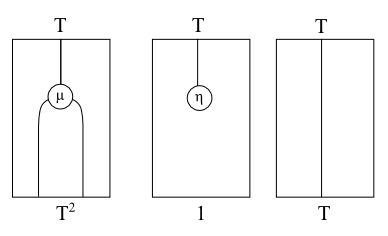The geometric intuition is that the monad laws are reflected as topological properties of the string diagrams. Both $$\mu$$ and $$\eta$$ exhibit reflection symmetry and that we topologically straighten out $$\eta$$ to yield the identity functor.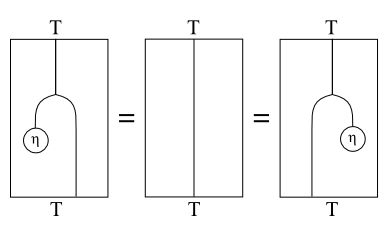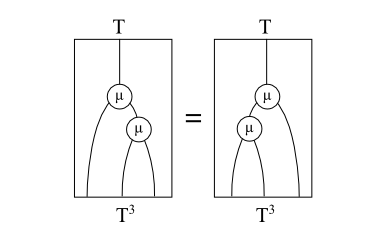In Haskell we can normally construct the Monad type class from an Endofunctor and ($$\mu, \eta$$) or join and return.

class (Functor t) => Monad t where
eta :: a -> (t a)
mu  :: (t (t a)) -> (t a)

(>>=) :: t a -> (a -> t b) -> t b
ma >>= f = mu . fmap f

return = eta
join = mu

What is not immediately apparent though is that every adjoint pair of functors gives rise to a monad $$(T, \mu, \eta)$$ over a category $$C$$ induced by the composition of the functors to give rise to a endofunctor and natural transformations in terms of the unit and counit of the underlying adjunction:

\begin{align} T &= G \circ F & : &C \rightarrow C \\ \mu &= G \epsilon & : &T^2 \rightarrow T \\ \end{align}

class Adjoint f g => Monad f g where
muM :: g (f (g (f a))) -> g (f a)
muM = fmap epsilon

etaM :: a -> g (f a)
etaM = eta

(>>=) :: g (f a) -> (a -> g (f b)) -> g (f b)
x >>= f = muM (fmap (fmap f) x)

The geometric intution for this is clear: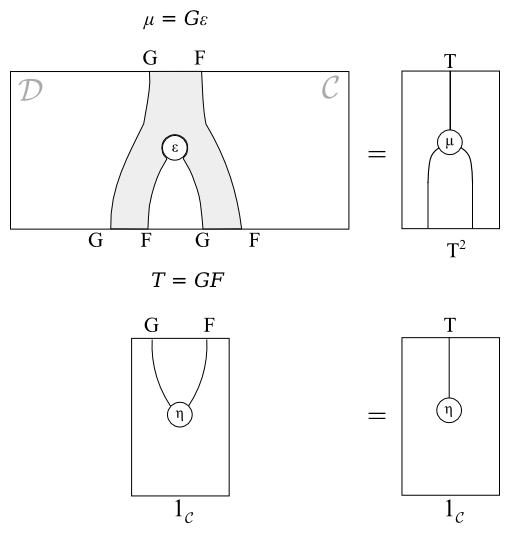From the Monad we can then construct the Kleisli category in the usual way.

class (Adjoint f g, Category c) => Kleisli c f g where
idK :: c x (g (f x))
(<=<) :: c y (g (f z)) -> c x (g (f y)) -> c x (g (f z))

instance Monad f g => Kleisli (->) f g where
idK = eta
g <=< f = muM . fmap (fmap g) . f

instance Kleisli c f g => Monoid (c a (g (f a))) where
mempty  = idK
mappend = (<=<)

In retrospect this is trivial, but importantly leads us to the more important question: Can we recover an adjunction from a monad. The answer is Yes…